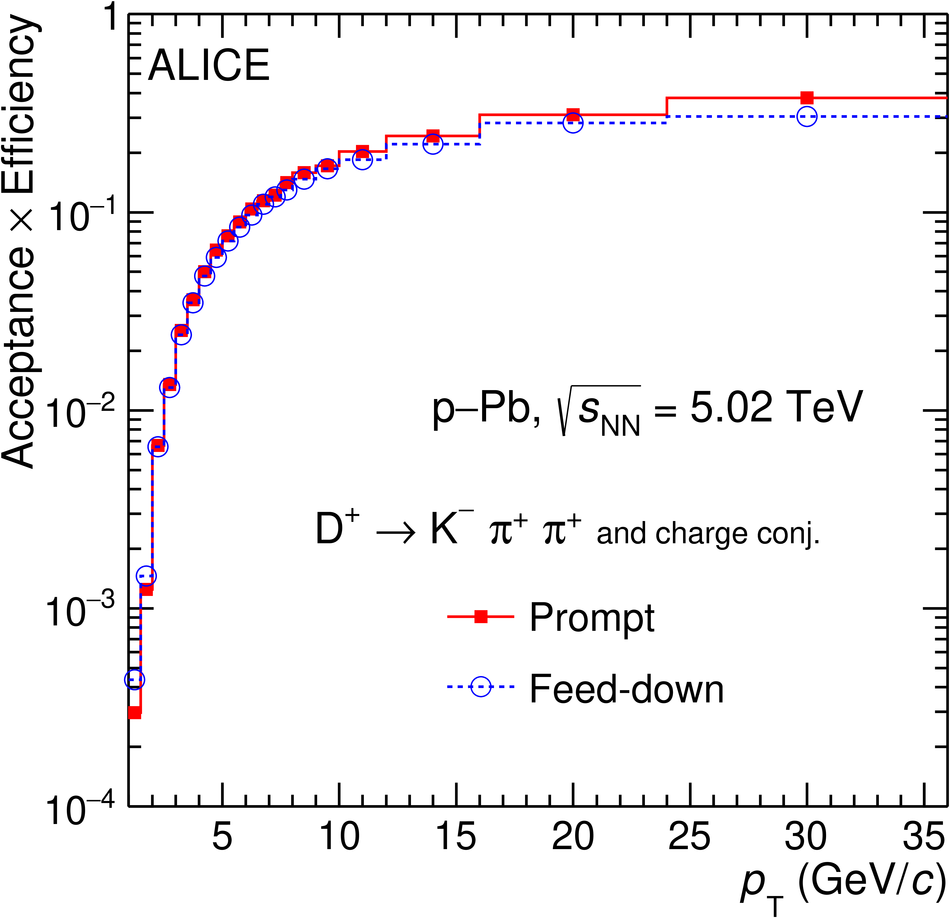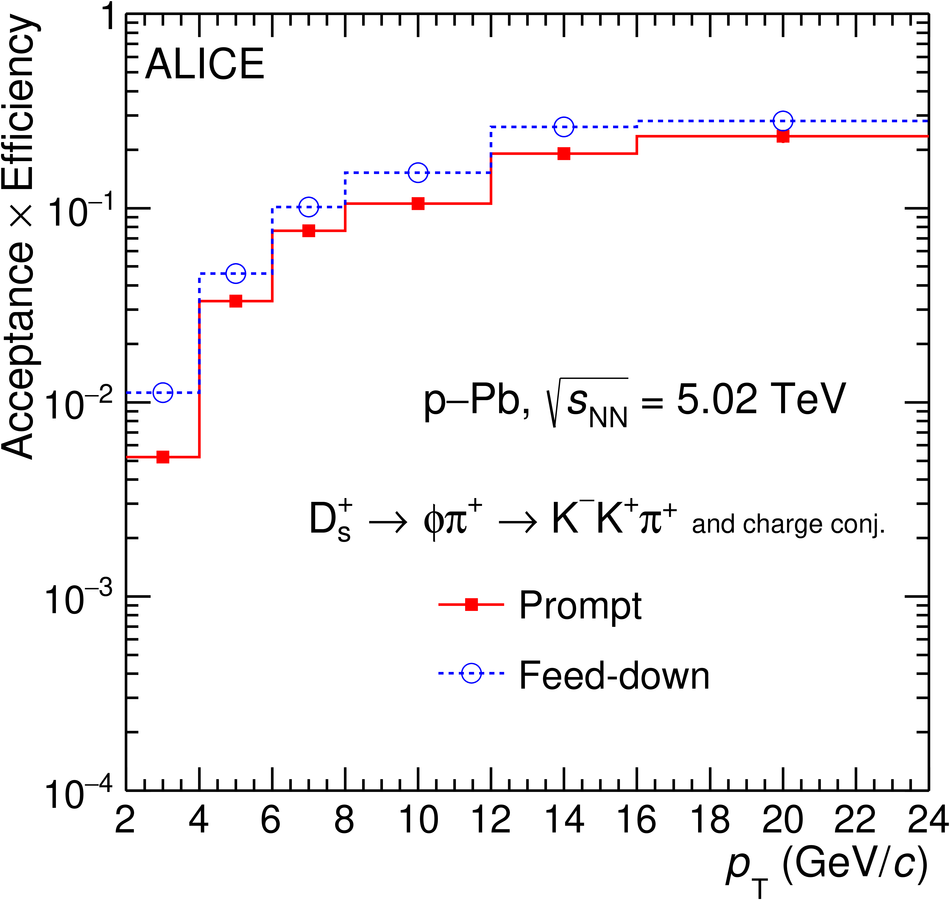# Figure 2

 The product of acceptance and efficiency for $\Dzero$, $\Dplus$, $\Dstar$, and $\Ds$ mesons as a function of transverse momentum in p--Pb collisions at $\sqrtsNN=5.02 \tev$ The values for prompt (solid line) and feed-down (dashed line) D mesons are shown